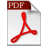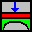Verification Examples of Analyzing Axisymmetric Structures and Tanks
ELPLA (ELastic PLAte) is a software which is used for analyzing geotechnical and structure problems. One of them is analyzing axisymmetric structure or tank with the real subsoil model.

• Three known subsoil models for the analysis of axisymmetric structures and tanks (standard models) are considered. The subsoil models are the Simple Assumption Model, the Winkler Model and the Continuum Model
• The mathematical solution of the structures is based on the FE-Method. The program can analyze different types of subsoil models, especially the Continuum model that considers any number of soil layers under the structure base
• A good advantage of this program is the capability to handle the three analyses of flexible, elastic and rigid bases. In addition, the mesh of the rigid and flexible bases can be constructed to be analogous to the finite elements mesh of the elastic foundation. Therefore, the three analyses can be compared easily and correctly• ELPLA can analyze different structures with different types of subsoil models
• In this textbook some problems published previously by researchers using different methods of analyses and models are compared with the results obtained by the analysis used in this textbook using ELPLA
• A verification study is carried out using ELPLA to analyze circular cylindrical tanks with different subsoil models
• The mathematical solution of the circular cylindrical tanks is based on the Finite Element Method using circular cylindrical shell elements
• Items to be checked under different conditions are internal forces, deformations and rotations in the tank wall and baseAnalysis of Axisymmetric Structures and Tanks by ELPLA Part II (Verification Examples)ELPLA-Data Files of Verification Examples.rar